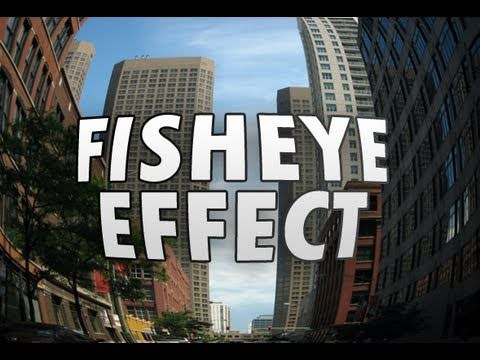# pouët.net

## What is the name of this effect?

category: code [glöplog]

What is the name of this effect: https://youtu.be/e-WWfNNAK5k?t=65

Please can you explain me how it works?
Basically 2D blobs where you use the final brightness and angle as a texture lookup. Not sure if there's a name aside from "table effect" I guess.
move.w (a2)+,d2
(...)
move.b (a1,d2.l),(a0)+
tunnel blobs
I've heard them called bitmap tunnels. Tutorial here: http://sol.gfxile.net/gp/ch17.html

Basically you have a table of distance-from-center and around-the-circle values which you use as texture u/v lookup values. Those tables can be made in different ways (the example you gave doesn't have a straight tunnel but has a sphere in the middle), and when you offset the tables and sum them together you get all sorts of fun things. You can also have one tunnel going in one way and another one in another, etc..
Great demo, and this effect looks more complicated than it actually is, or an 060 would be required to process it. As for the artwork, I always loved Cyclone's "Garden of Eden" picture in this prod.
i am sorry for the dumb question but the video is pointing at 1:05 where there are some spheres that look like a polar coordinates plasma is mapped onto them including lighting?

i don't see a tunnel here?It's still a tunnel for some definition of a tunnel :) The table is just different and doesn't contain a perspective.

Pseudocode for this effect:

Code:``` for (int y = 0; y < screenHeight; y++) { for (int x 0; x < screenWidth; x++) { int u = 0; int v = 0; for (int i = 0; i < numTunnels; i++) { //tunnels[] contains center points int centerX = tunnels[i].x; int centerY = tunnels[i].y; //the tunnel table is for example twice the size of the screen in both dimensions //we assume the coordinates are sensible so we don't read out of the screen int offsetToTable = (x + centerX) + (y + centerY) * tableWidth; u += table1[offsetToTable]; v += table2[offsetToTable]; } //classic 256x256 texture int pixel = texture[(u & 255) + (v & 255) * textureWidth]; //shading is left as an exercies for the reader :) screen[x + y * screenWidth] = pixel; } } ```

(might contain bugs, it's been like 20 years...)Well, you'll need some offset coordinates to make the darn texture-coordinates move like "flux-lines" in an electric field (though sort of fake simulated), and then the lens-effect on top of that.
If it is done like 2d-blobs, then of course its easy-peasy to just final = blob1+blob2+blob3. and then pixel = texture[final]..
There is also distortion outside of blobs, so you do something like z^2 = abs(r - x^2 + y^2) and it wraps upwards. So, you have the wrap outside the sphere like here https://youtu.be/h6pF4gIAsuI?t=119. Then you can add three of these maybe.
abs(r - x^2 - y^2)
How are 2D blobs done fast anyway?
exponential tunnel!
Quote: